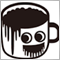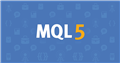• Get access

# Move stoploss to breakeven. Not working.304

Hello World!

Could anyone give me some advice or a clue on how to get my breakeven function to work?

Not asking for anyone to fix my code as I respect you all and there are no slaves here. :)

Here is my attempt.

Just trying to create a function that will move the stop-loss to break-even.

Pretty sure not all the code is working as intended. Just trying to fix this part first haha.

I will post all the code so you can see the whole picture.

Thanks in advance for anyone willing to point me in the right direction. And yes I have read the manual. It just doesnt always stay in my brain ^^

```//Input Variables (F7)

input int         MagicNumber      = 222001;   // Magic Number
input int         MaximumTrades    = 4;        // Maximum Permitted Active Trades & Orders
input int         MinSellGap       = 40;
input int         MaxSellGap       = 121;
input int         EntryGap         = 30;
input double      MaxRiskPerTrade  = 1.0;      //% of balance to risk in one trade
input double      BreakEvenGap     = 30;
input double      BreakEvenTrigger = 60;
input double      StopLoss         = 200;
input double      TakeProfit       = 200;

bool    TestPrint = false;
int     OrderCount;
double  CalcLotSz;

//input string      ATRexp = "this function to calculate stoploss depend on iATR";
//input bool        ATR = true;
//input int         ATRMultiplier = 3;

string Order_Comment;
string Chart_Period;

//+------------------------------------------------------------------+
//| StopLoss Calculations                                            |
//+------------------------------------------------------------------+
/*
double StpLoss(int i) {

double SL=0;

if(StopLoss==0 && !ATR) SL=0;

else if(StopLoss>0 && !ATR) {

double MyPoint=Point;
if(Digits==3 || Digits==5) MyPoint=Point*10;
if(i==1) SL=Bid+(StopLoss*MyPoint);
}

else if(ATR) {

double AvTR=iATR(Symbol(),30,14,0);
if(i==1) SL=Bid+(ATRMultiplier*AvTR);
}

return(SL);
// for market excuting orders Buy(i=0)-->(StpLoss(0)) / Sell(i=1)-->(StpLoss(1))
// OR for OrderModify() you can put StopLoss= StpLoss(OrderType())
}
*/
//+-------------------------------------------------------------------+
//+ Calculate the position size and return the lot to order           |
//+                                                                   |
//+ Parameter 1 - parsed available amount (Balance/Equity/Free Margin |
//+ Parameter 2 - Stop Loss                                           |
//+                                                                   |
//+ Return      - Calclated Lotsize                                   |
//+-------------------------------------------------------------------+

double CalcLotSize(double TotalAmount, double SL) {

double LotSize = 0;
// Get the value of a tick
double nTickValue=MarketInfo(Symbol(),MODE_TICKVALUE);

// Formula to calculate the position size and assign the value to the variable
LotSize = LotSize - 0.005;       // Round Up

//Print("Lotsize  ",LotSize);
if(LotSize<0.01) LotSize=0.01;
if(LotSize>0.01 && LotSize<1.0) LotSize=NormalizeDouble(LotSize,2);
if(LotSize>0.1 && LotSize<10.0) LotSize=NormalizeDouble(LotSize,2);
if(LotSize>10.0) LotSize=NormalizeDouble(LotSize,2);
if (TestPrint) {

Print("+++++++++++++++++ nTickValue - ",DoubleToStr(nTickValue,8));
Print("+++++++++++++++++ LotSize - ",DoubleToStr(LotSize,8));
TestPrint = false;
}

//LotSize=MathRound(LotSize/MarketInfo(Symbol(),MODE_LOTSTEP))*MarketInfo(Symbol(),MODE_LOTSTEP);
return LotSize;
}

//+------------------------------------------------------------------+
//| Magic Number Order Count                                         |
//|                                                                  |
//| Count open trades & orders for Currency Pair & this timeframe    |
//+------------------------------------------------------------------+

int k;
int OCt = 0;

for(k = OrdersTotal() - 1; k >= 0; k--) {

if(OrderSymbol() == Symbol() && OrderMagicNumber() == MagicNumber) {

OCt = OCt + 1;
}
}
}

return(OCt);
}

//+------------------------------------------------------------------+
//| Start function                                                   |
//+------------------------------------------------------------------+

void start() {

CalcLotSz = CalcLotSize(AccountBalance(),StopLoss);

//Print("CalcLotSize - "+CalcLotSize);

CheckPositions();       // Check for Open Order Positions
}
}

//+------------------------------------------------------------------+
//| Move StopLoss to Break-even                                      |
//+------------------------------------------------------------------+

void MoveToBreakeven() {

// Set the Break-even position for long or short orders.
double BreakEvenLongPos   = OrderOpenPrice() + (BreakEvenGap*Point);
double BreakEvenShortPos  = OrderOpenPrice() - (BreakEvenGap*Point);
int    i;

// Create a loop for checking orders.
for (i = OrdersTotal() - 1; i >= 0; i--) {

if(OrderSymbol() == Symbol() && OrderMagicNumber() == MagicNumber) {

switch(OrderType()) {

// This makes sure the quote data is up to date.
RefreshRates();

// Modifies Stop Loss price for buy orders
if (Ask + (BreakEvenGap*Point) > OrderOpenPrice() + (BreakEvenTrigger*Point)) {

bool ModifyLong = OrderModify(OrderTicket(),OrderOpenPrice(),BreakEvenLongPos,OrderTakeProfit(),0,Blue);

if(!ModifyLong) {

Print("Error in OrderModify. Error code=",GetLastError());
}

else {

Print("Order modified successfully.");
}
}

break;

case OP_SELL:

RefreshRates();

// Modifies Stop Loss price for Short orders
if (Bid - (BreakEvenGap*Point) < OrderOpenPrice() - (BreakEvenTrigger*Point)) {

bool ModifyShort = OrderModify(OrderTicket(),OrderOpenPrice(),BreakEvenShortPos,OrderTakeProfit(),0,Blue);

if(!ModifyShort) {

Print("Error in OrderModify. Error code=",GetLastError());
}

else {

Print("Order modified successfully.");
}
}

break;

//default:
}
}
}
}
}

//+------------------------------------------------------------------+
//| Check for open order conditions                                  |
//+------------------------------------------------------------------+

void CheckPositions() {

// Indicators
double UpperBand = iBands(NULL,0,20,2,0,PRICE_LOW,MODE_UPPER,0);
double LowerBand = iBands(NULL,0,20,2,0,PRICE_LOW,MODE_LOWER,0);

int ticket;

RefreshRates(); // Update Bid and Ask value.

if (ticket < 0) {

// Error = GetLastError();
Print("OrderSend Error ",ticket," ",DoubleToStr(CalcLotSz,Digits));
}

else {

LastTrade = Time; // Once per bar
}
}

// Sell conditions
if (Time != LastTrade && Bid > UpperBand) {

ticket=OrderSend(Symbol(),OP_SELL,CalcLotSz,Bid,3,Bid+(StopLoss*Point),Bid-(TakeProfit*Point),Order_Comment,MagicNumber,0,Red);

if (ticket < 0) {

// Error = GetLastError();
Print("OrderSend Error ",ticket," ",DoubleToStr(CalcLotSz,Digits));
}

else {

LastTrade = Time; // Once per bar
}
}

return;
}
//+------------------------------------------------------------------+```Documentation on MQL5: Constants, Enumerations and Structures / Named Constants / Predefined Macro Substitutions
• www.mql5.com
//| Expert initialization function                                   | //| Expert deinitialization function                                 | //| Expert tick function                                             | //| test1                                                            |...20384

Use the debugger or print out your variables, including _LastError and prices and . Do you really expect us to debug your code for you?304

William Roeder:
Use the debugger or print out your variables, including _LastError and prices and . Do you really expect us to debug your code for you?

If you actually read my message you would know I am not asking for anyone to debug my code.

May I ask, if someone were to ask for some help on here based on my question, how should they construct their question to avoid a response like yours?

I was not trying to make anyone do any work for me. I was asking for advice.20384

1. Kyle Paarman: Pretty sure not all the code is working as intended. Thanks in advance for anyone willing to point me in the right direction.

The right direction is debugging your code.

2. ```   double BreakEvenLongPos   = OrderOpenPrice() + (BreakEvenGap*Point);
double BreakEvenShortPos  = OrderOpenPrice() - (BreakEvenGap*Point);
```
You can not use any Trade Functions unless you select an order first.

3. ```      ticket=OrderSend(Symbol(),OP_BUY,CalcLotSz,Ask,3,Ask-(StopLoss*Point),Ask+(TakeProfit*Point),Order_Comment,MagicNumber,0,Red);

ticket=OrderSend(Symbol(),OP_SELL,CalcLotSz,Bid,3,Bid+(StopLoss*Point),Bid-(TakeProfit*Point),Order_Comment,MagicNumber,0,Red);

if (Ask + (BreakEvenGap*Point) > OrderOpenPrice() + (BreakEvenTrigger*Point)) {

if (Bid - (BreakEvenGap*Point) < OrderOpenPrice() - (BreakEvenTrigger*Point)) {
```
You buy at the Ask and sell at the Bid. So for buy orders you pay the spread on open. For sell orders you pay the spread on close.
1. Your buy order's TP/SL (or Sell Stop's/Sell Limit's entry) are triggered when the Bid reaches it. Not the Ask. Your SL is shorter by the spread and your TP would be longer. Don't you want the same/specified amount for either direction?
MODE_SPREAD (Paul) - MQL4 programming forum - Page 3 #25
3. The charts show Bid prices only. Turn on the Ask line to see how big the spread is (Tools → Options (Control-O) → charts → Show ask line.)

4. ```   LotSize=(TotalAmount*MaxRiskPerTrade/100)/(SL*nTickValue);
```
Never risk more than a small percentage of your account, certainly less than 2% per trade, 6% total to the account. Risk depends on your initial stop loss, lot size, and the value of the pair. It does not depend on margin and leverage.
1. You place the stop where it needs to be — where the reason for the trade is no longer valid. E.g. trading a support bounce the stop goes below the support.
2. AccountBalance * percent/100 = RISK = OrderLots * (|OrderOpenPrice - OrderStopLoss| * DeltaPerLot + CommissionPerLot) (Note OOP-OSL includes the spread, and DeltaPerLot is usually around \$10/pip but it takes account of the exchange rates of the pair vs. your account currency.)
3. Do NOT use TickValue by itself - DeltaPerLot and verify that MODE_TICKVALUE is returning a value in your deposit currency, as promised by the documentation, or whether it is returning a value in the instrument's base currency.
MODE_TICKVALUE is not reliable on non-fx instruments with many brokers - MQL4 programming forum 2017.10.10
Is there an universal solution for Tick value? - Currency Pairs - General - MQL5 programming forum 2018.02.11
Lot value calculation off by a factor of 100 - MQL5 programming forum 2019.07.19
4. You must normalize lots properly and check against min and max.
5. You must also check FreeMargin to avoid stop out

Most pairs are worth about \$10 per PIP. A \$5 risk with a (very small) 5 PIP SL is \$5/\$10/5 or 0.1 Lots maximum.